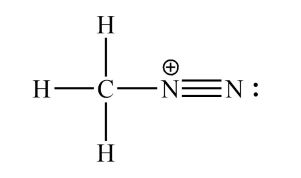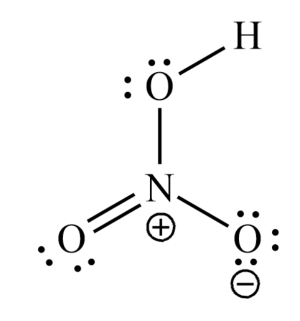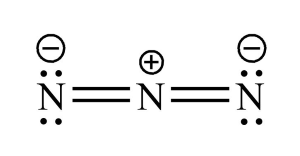# Draw a Lewis structure for each of the following. a. {eq}CH_3N_2^+ {/eq} b. {eq}HNO_3 {/eq} c. {eq}N_3^- {/eq} d. {eq}O_3 {/eq}

## Question:

Draw a Lewis structure for each of the following.

a. {eq}CH_3N_2^+ {/eq}

b. {eq}HNO_3 {/eq}

c. {eq}N_3^- {/eq}

d. {eq}O_3 {/eq}

## Ozone:

Ozone is an inorganic molecule that is denoted by the chemical formula {eq}{O_3} {/eq}. It is an allotropic form of oxygen. Generally, ozone is present in the troposphere as a layer that helps in the protection of the earth from harmful UV radiations.

Become a Study.com member to unlock this answer!

Explanation

Lewis's structures are represented as follows:

{eq}a) C{H_3}N_2^ + {/eq}{eq}b) HN{O_3} {/eq}{eq}c) N_3^ - {/eq}{...